# Low Cycle Fatigue

Low cycle fatigue (LCF) usually refers to situations where the stress is high enough for plastic deformation to occur, the accounting of the loading in terms of stress is less useful and the stain in the material offers a simpler and more accurate description. This type of fatigue is normally experienced by components which undergo elastic-plastic or plastic deformation.  The reason why strain life is more suitable for low cycle fatigue is that the strains allow the elastic and plastic components to be distinguished from each other, whereas the stress is way less sensitive to the plastic component in the case of high amplitude.  The arbitrary classification between high cycle fatigue (HCF) and low cycle fatigue is considered to be roughly 10,000 cycles; however, the exact number of cycles really depends on the properties of the metal.The research of low cycle fatigue was traditionally done for boilers, steam turbines, gas turbines, pressure vessels, nuclear reactors and other power equipment that are exposed to high temperatures which induce thermal stresses in the components.

Coffin-Manson Relation

Low-cycle fatigue is usually characterized by the Coffin-Manson relation (published independently by L. F. Coffin in 1954 and S. S. Manson in 1953):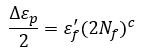where,

• Δεp /2 is the plastic strain amplitude
• 2Nf is the number of reversals to failure (Nf cycles)
• εf‘ is an empirical constant known as the fatigue ductility coefficient, the failure strain for a single reversal. A fairly good approximation is ε’f = εf with fracture ductility εf = ln[100/(100 – RA)], where RA is the reduction of area in %. Other values in estimating ε’f include:

ε’f = 0.35 εf
ε’f = 0.5 εf (c = -0.6)
ε’f = 0.75 εf0.75 (c = -0.6)
ε’f = 0.76 εf0.6 (c = -0.6)
ε’f = 0.50 εf
ε’f = 0.71 εf

• c is an empirical constant known as the fatigue ductility exponent, commonly ranging from -0.5 to -0.7 for metals in time independent fatigue.  Fairly ductile metals (say εf = 1.0) have value of c = -0.6; for strong metals (say εf = 0.5) a value of c = -0.5 is more reasonable. Slopes can be considerably steeper in the presence of creep or environmental interactions

Basquin’s Equation

The Coffin-Manson formula describes the relationship between plastic strain and fatigue life in the low-cycle high-strain fatigue regime. Basquin’s equation, on the other hand, describes high-cycle low strain behavior in elastic nature: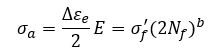or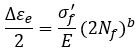where

• σa is the elastic stress amplitude
• Δεe /2 is the elastic strain amplitude
• 2Nf is the number of reversals to failure (Nf cycles)
• E is modulus of elasticity
• σ’f is fatigue strength coefficient.  A fairly good approximation is σ’f = σf where σf is fracture stress with necking corrections.  For steels with hardness below 500 HB, σf = UTS + 50 ksi. Other values in estimating σ’f include:

σ’f = 1.09 σf
σ’f = 0.92 σf (b = -0.12)
σ’f = 1.15 σf (b = -0.12)

• b is the fatigue strength exponent, commonly in the range -0.12 < b < -0.05 with an average of -0.085

The Fatigue+ software provides a material database including extensive fatigue data for carbon steels, low alloy steels, high alloy steels, aluminum alloys, as well as cast metals and welds.  The user can edit the database or add materials to the database.

Morrow Design Rule (Basquin-Coffin-Manson relation)

The Coffin-Manson formula describes the relationship between plastic strain and fatigue life in the low-cycle high-strain fatigue regime. Basquin’s equation describes high-cycle low strain behavior, as discussed above.  Morrow combines elastic strain and plastic strain into a total strain relationship as follows:The figure below shows the elastic, plastic and total strain fatigue curves.  The strain fatigue curves are also called E-N curves as opposed to S-N curves for stress fatigue curves.  The elastic strain curve represents Basquin’s equation; the plastic strain curve represents Coffin-Manson’s equation; and the total strain curve represents Morrow’s equation.  Notice from the figure that total strain is the sum of elastic strain and plastic strain.  The intersection of elastic and plastic curves is denoted as 2Nt, which is the demarcation of material behaviors.  The first half of the total strain fatigue curve on the left with higher slope represents low cycle fatigue (LCF); the second half on the right with the lower slope pertains to lower strain ranges that impart stresses in the elastic regime, which represents the high cycle fatigue (HCF).The following chart is an example of the strain fatigue curves generated by the Fatigue+ software.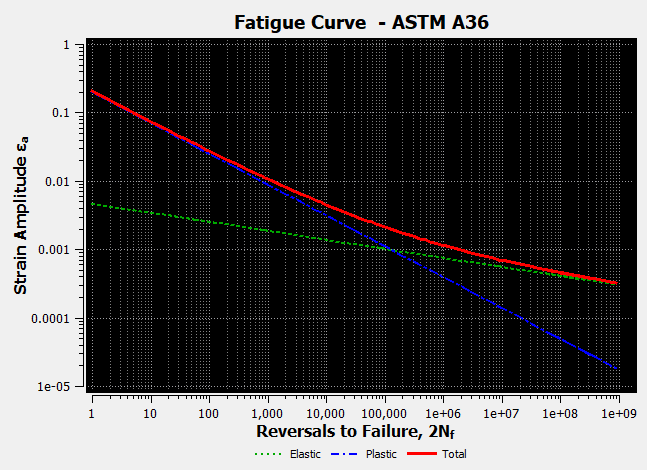Fatigue curve of ASTM A36

Elastic – Basquin’s equation

Plastic – Coffin-Manson’s equation

Total – Morrow’s equation

### Mean Stress Correction

High cycle fatigue is sensitive to mean stresses.  In the software Fatigue+, the following options are available for mean stress correction:

For Basquin model, select Soderberg, Goodman, Gerber,Morrow, Smith-Watson-Topper, or None. (See High Cycle Fatigue)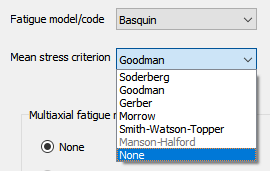For Basquin-Coffin-Manson relation, select Morrow, Smith-Watson-Topper, Manson-Halford, or None.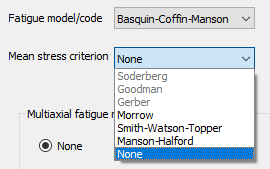In the design code based fatigue models, the mean stress correction is often incorporated into the models in compliance with corresponding design codes.